Next: The 1-d advection equation Up: The wave equation Previous: The wave equation

## Introduction

The wave equation, which in one dimension takes the form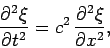(230)

occurs so frequently in physics that it is not necessary to enumerate examples. Here,is usually some sort of displacement or perturbation, whereas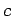is the (constant) wave speed. The wave equation possesses the formal solution(231)

where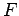and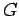are arbitrary functions. The above solution represents arbitrarily shaped wave pulses propagating with speedin theand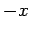directions, respectively, without changing shape.

The wave equation, which is second-order in space and time, can be written as two coupled first-order equations by defining the new variables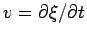and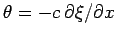. Expressing Eq. (230) in terms of these new variables, we obtain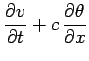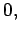(232)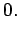(233)

Note that when solving the wave equation numerically it is generally preferable to write it as a set of coupled first-order equations, as shown above.Next: The 1-d advection equation Up: The wave equation Previous: The wave equation
Richard Fitzpatrick 2006-03-29